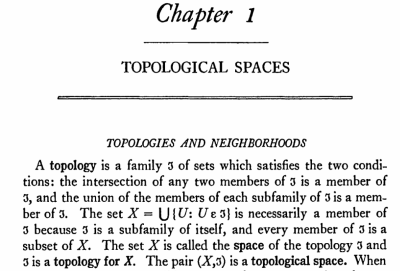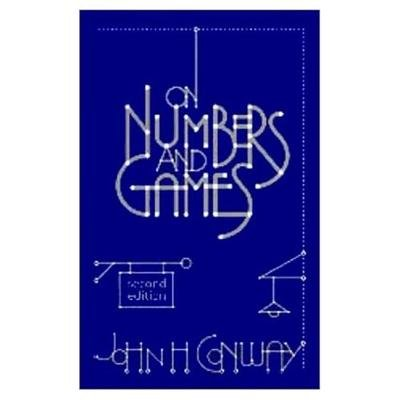# The Universe of Discourse

Fri, 24 Aug 2012

More about ZF's asymmetry between union and intersection
In an article earlier this week, I explored some oddities of defining a toplogy in terms of closed sets rather than open sets, mostly as a result of analogous asymmetry in the ZF set theory axioms.

Let's review those briefly. The relevant axioms concern the operations by which sets can be constructed. There are two that are important. First is the axiom of union, which says that if !!{\mathcal F}!! is a family of sets, then we can form !!\bigcup {\mathcal F}!!, which is the union of all the sets in the family.

The other is actually a family of axioms, the specification axiom schema. It says that for any one-place predicate !!\phi(x)!! and any set !!X!! we can construct the subset of !!X!! for which !!\phi!! holds:

$$\{ x\in X \;|\; \phi(x) \}$$

Both of these are required. The axiom of union is for making bigger sets out of smaller ones, and the specification schema is for extracting smaller sets from bigger ones. (Also important is the axiom of pairing, which says that if !!x!! and !!y!! are sets, then so is the two-element set !!\{x, y\}!!; with pairing and union we can construct all the finite sets. But we won't need it in this article.)

Conspicuously absent is an axiom of intersection. If you have a family !!{\mathcal F}!! of sets, and you want a set of every element that is in some member of !!{\mathcal F}!!, that is easy; it is what the axiom of union gets you. But if you want a set of every element that is in every member of !!{\mathcal F}!!, you have to use specification.

Let's begin by defining this compact notation: $$\bigcap_{(X)} {\mathcal F}$$

for this longer formula: $$\{ x\in X \;|\; \forall f\in {\mathcal F} . x\in f \}$$

This is our intersection of the members of !!{\mathcal F}!!, taken "relative to !!X!!", as we say in the biz. It gives us all the elements of !!X!! that are in every member of !!{\mathcal F}!!. The !!X!! is mandatory in !!\bigcap_{(X)}!!, because ZF makes it mandatory when you construct a set by specification. If you leave it out, you get the Russell paradox.

Most of the time, though, the !!X!! is not very important. When !!{\mathcal F}!! is nonempty, we can choose some element !!f\in {\mathcal F}!!, and consider !!\bigcap_{(f)} {\mathcal F}!!, which is the "normal" intersection of !!{\mathcal F}!!. We can easily show that $$\bigcap_{(X)} {\mathcal F}\subseteq \bigcap_{(f)} {\mathcal F}$$ for any !!X!! whatever, and this immediately implies that $$\bigcap_{(f)} {\mathcal F} = \bigcap_{(f')}{\mathcal F}$$ for any two elements of !!{\mathcal F}!!, so when !!{\mathcal F}!! contains an element !!f!!, we can omit the subscript and just write $$\bigcap {\mathcal F}$$ for the usual intersection of members of !!{\mathcal F}!!.

Even the usually troublesome case of an empty family !!{\mathcal F}!! is no problem. In this case we have no !!f!! to use for !!\bigcap_{(f)} {\mathcal F}!!, but we can still take some other set !!X!! and talk about !!\bigcap_{(X)} \emptyset!!, which is just !!X!!.

Now, let's return to topology. I suggested that we should consider the following definition of a topology, in terms of closed sets, but without an a priori notion of the underlying space:

A co-topology is a family !!{\mathcal F}!! of sets, called "closed" sets, such that:

1. The union of any two elements of !!{\mathcal F}!! is again in !!{\mathcal F}!!, and
2. The intersection of any subfamily of !!{\mathcal F}!! is again in !!{\mathcal F}!!.
Item 2 begs the question of which intersection we are talking about here. But now that we have nailed down the concept of intersections, we can say briefly and clearly what we want: It is the intersection relative to !!\bigcup {\mathcal F}!!. This set !!\bigcup {\mathcal F}!! contains anything that is in any of the closed sets, and so !!\bigcup {\mathcal F}!!, which I will henceforth call !!U!!, is effectively a universe of discourse. It is certainly big enough that intersections relative to it will contain everything we want them to; remember that intersections of subfamilies of !!{\mathcal F}!! have a maximum size, so there is no way to make !!U!! too big.

It now immediately follows that !!U!! itself is a closed set, since it is the intersection !!\bigcap_{(U)} \emptyset!! of the empty subfamily of !!{\mathcal F}!!.

If !!{\mathcal F}!! itself is empty, then so is !!U!!, and !!\bigcap_{(U)} {\mathcal F} = \emptyset!!, so that is all right. From here on we will assume that !!{\mathcal F}!! is nonempty, and therefore that !!\bigcap {\mathcal F}!!, with no relativization, is well-defined.

We still cannot prove that the empty set is closed; indeed, it might not be, because even !!M = \bigcap {\mathcal F}!! might not be empty. But as David Turner pointed out to me in email, the elements of !!M!! play a role dual to the extratoplogical points of a topological space that has been defined in terms of open sets. There might be points that are not in any open set anywhere, but we may as well ignore them, because they are topologically featureless, and just consider the space to be the union of the open sets. Analogously and dually, we can ignore the points of !!M!!, which are topologically featureless in the same way. Rather than considering !!{\mathcal F}!!, we should consider !!{\widehat{\mathcal F}}!!, whose members are the members of !!{\mathcal F}!!, but with !!M!! subtracted from each one:

$${\widehat{\mathcal F}} = \{\hat{f}\in 2^U \;|\; \exists f\in {\mathcal F} . \hat{f} = f\setminus M \}$$

So we may as well assume that this has been done behind the scenes and so that !!\bigcap {\mathcal F}!! is empty. If we have done this, then the empty set is closed.

Now we move on to open sets. An open set is defined to be the complement of a closed set, but we have to be a bit careful, because ZF does not have a global notion of the complement !!S^C!! of a set. Instead, it has only relative complements, or differences. !!X\setminus Y!! is defined as: $$X\setminus Y = \{ x\in X \;|\; x\notin Y\}$$

Here we say that the complement of !!Y!! is taken relative to !!X!!.

For the definition of open sets, we will say that the complement is taken relative to the universe of discourse !!U!!, and a set !!G!! is open if it has the form !!U\setminus f!! for some closed set !!f!!.

Anatoly Karp pointed out on Twitter that we know that the empty set is open, because it is the relative complement of !!U!!, which we already know is closed. And if we ensure that !!\bigcap {\mathcal F}!! is empty, as in the previous paragraph, then since the empty set is closed, !!U!! is open, and we have recovered all the original properties of a topology.

 BuyGeneral Topologyfrom Bookshop.org(with kickback)(without kickback)
But gosh, what a pain it was; in contrast recovering the missing axioms from the corresponding open-set definition of a topology was painless. (John Armstrong said it was bizarre, and probably several other people were thinking that too. But I did not invent this bizarre idea; I got it from the opening paragraph of John L. Kelley's famous book General Topology, which has been in print since 1955.Here Kelley deals with the empty set and the universe in two sentences, and never worries about them again. In contrast, doing the same thing for closed sets was fraught with technical difficulties, mostly arising from ZF. (The exception was the need to repair the nonemptiness of the minimal closed set !!M!!, which was not ZF's fault.)

 BuyOn Numbers and Gamesfrom Bookshop.org(with kickback)(without kickback)
I don't think I have much of a conclusion here, except that whatever the advantages of ZF as a millieu for doing set theory, it is overrated as an underlying formalism for actually doing mathematics. (Another view on this is laid out by J.H. Conway in the Appendix to Part Zero of On Numbers and Games (Academic Press, 1976).) None of the problems we encountered were technically illuminating, and nothing was clarified by examining them in detail.

On the other hand, perhaps this conclusion is knocking down a straw man. I think working mathematicians probably don't concern themselves much with whether their stuff works in ZF, much less with what silly contortions are required to make it work in ZF. I think day-to-day mathematical work, to the extent that it needs to deal with set theory at all, handles it in a fairly naïve way, depending on a sort of folk theory in which there is some reasonably but not absurdly big universe of discourse in which one can take complements and intersections, and without worrying about this sort of technical detail.

[ MathJax doesn't work in Atom or RSS syndication feeds, and can't be made to work, so if you are reading a syndicated version of this article, such as you would in Google Reader, or on Planet Haskell or PhillyLinux, you are seeing inlined images provided by the Google Charts API. The MathJax looks much better, and if you would like to compare, please visit my blog's home site. ]